Complex Sinusoids Next  |  Prev  |  Up  |  Top  |  Index  |  JOS Index  |  JOS Pubs  |  JOS Home  |  Search

## Complex Sinusoids

Recall Euler's Identity,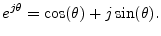Multiplying this equation by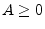and setting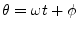, where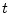is time in seconds,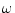is radian frequency, and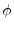is a phase offset, we obtain what we call the complex sinusoid: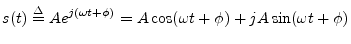Thus, a complex sinusoid consists of an in-phase'' component for its real part, and a phase-quadrature'' component for its imaginary part. Since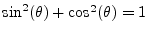, we have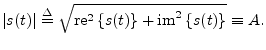That is, the complex sinusoid has a constant modulus (i.e., a constant complex magnitude). (The symbol'' means identically equal to,'' i.e., for all.) The instantaneous phase of the complex sinusoid is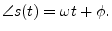The derivative of the instantaneous phase of the complex sinusoid gives its instantaneous frequency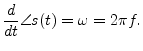Subsections
Next  |  Prev  |  Up  |  Top  |  Index  |  JOS Index  |  JOS Pubs  |  JOS Home  |  Search

[How to cite this work] [Order a printed hardcopy]